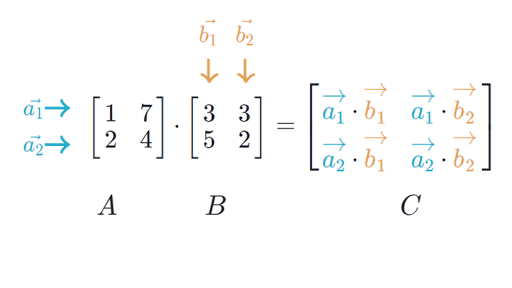A lot of content summarized from Mark Schmidt’s notes on Linear Algebra

## Notation §

Generally column major

• Scalar (1,1):
• Column Vector (m, 1):
• Row Vector (1, n):
• Matrix (m, n):

## Operations §

### Transpose §

A matrix is symmetric if

Associative (brackets don’t matter) and commutative (order independent)

### Scalar Multiplication §

Associative (brackets don’t matter) and commutative (order independent)

### Inner Product §

Between two vectors of the same length, multiply each element together to get a scalar result

A specific version of this is the dot product which can be expressed as the inner product between two vectors,

• Commutative:

### Outer Product §

Between two vectors of the same length, create a matrix multiplying each combination of elements in each vector.

Given two vectors and ,

The resulting matrix is always rank-1.

### Multiplication §

In general, we can multiply matrices A and B when the number of columns in A matches the number of rows in B

If A is (m, n) and B is (n, p), then AB is (m, p)• Associative:
• Generally not commutative:
• Transposing reverses order:
• Matrix powers don’t change order:
• Matrix-vector multiplication always yields a vector:

## Properties §

### Vector Norm §

A scalar measure of a vector’s length

• Euclidean Norm (L2-Norm):

• Also note that
• Manhattan Distance (L1-Norm):

• How many ‘blocks’ you need to traverse
• L-Norm:

• How many blocks you have to walk in any one dimensions

### Rank §

• The dimension of the vector space generated (or spanned) by its columns.
• This corresponds to the number of linearly independent columns of A.
• This minimal set of vectors that span a space is called a basis

### Orthogonal §

If for some set of vectors :

• , we call and orthogonal
• , we call and orthonormal

Inner product of square orthogonal matrices is the identity matrix:

### Linear Dependence §

A vector is linearly dependent on a set of vectors if it can be written as a linear combination of them

A set of vectors is linearly dependent if and only if the zero vector can be written as a non-trivial combination of any of the vectors.

A matrix with fully independent columns has full column rank. If this is the case, implies that

## Special Matrices §

### Identity Matrix §

1’s on the diagonal and 0’s otherwise. denotes an (n,n) identity matrix.

Multiplication by the identity matrix yields the original matrix. Columns of the identity matrix are called elementary vectors.

## Spaces §

### Range (Column-space) §

Subspace spanned by the columns of a matrix.

A system is solvable if and only if b is in ’s column-space

### Subspace §

A non-empty subset of vector space that is closed under addition and scalar multiplication

Possible spaces of

• 0 Vector
• Any line or plane through the origin
• All of

We say that the vectors generate or span the subspace when you can reach any point in the subspace through a linear combination of the vectors.

## Matrices as transformation §

Viewing

A linear transformation can’t move the origin. But, if there are linearly dependent columns, there are non-zero vectors that can be transformed to zero. The set of vectors that can be transformed to 0 is called the null-space.

Null space: is all such that

### Fundamental Theorem of Linear Algebra §

• is the dimension of the column-space which is the same as the dimension of the row-space
• The null-space is orthogonal to the row-space

## Inverses §

We can find the inverses if and only if A is square and doesn’t have null-space outside of the zero vector (otherwise we either lose information to the null-space or can’t get to all vectors)

If the inverse exists, it is a unique matrix such that

Some identities

• Assuming both and exist,

Special inverses of diagonal matrices

## Solving Linear Equations §

Given A and b, we want to solve for x in

Say, .

We can interpret this multiple ways:

1. By rows: is the intersection of the hyperplanes and
2. By columns: is the linear combination that yields the RHS in
3. Transformation

generally has a solution when is in the column-space of A. It has a single unique solution if the columns of A are linearly independent.

If has as solution we say it is consistent.

Basically,

We can solve using Gaussian Elimination